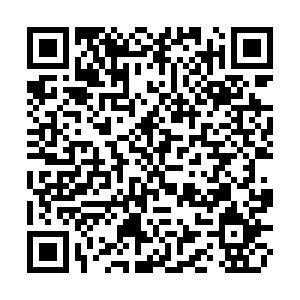## 留言板引用本文: 陈佳美, 李世昂, 李玉峰, 王宇鹏, 别玉霞. 基于甲虫搜索的改进粒子群无人机辅助网络部署优化算法[J]. 电子与信息学报, 2023, 45(5): 1697-1705.CHEN Jiamei, LI Shiang, LI Yufeng, WANG Yupeng, BIE Yuxia. Improved Particle Swarm Optimization Unmanned Aerial Vehicle-assisted Network Deployment Optimization Algorithm Based on Beetle Antennae Search[J]. Journal of Electronics & Information Technology, 2023, 45(5): 1697-1705. doi: 10.11999/JEIT220404
 Citation: CHEN Jiamei, LI Shiang, LI Yufeng, WANG Yupeng, BIE Yuxia. Improved Particle Swarm Optimization Unmanned Aerial Vehicle-assisted Network Deployment Optimization Algorithm Based on Beetle Antennae Search[J]. Journal of Electronics & Information Technology, 2023, 45(5): 1697-1705.## 基于甲虫搜索的改进粒子群无人机辅助网络部署优化算法

##### doi: 10.11999/JEIT220404

###### 通讯作者: 陈佳美　chenjiamei5870@163.com
• 中图分类号: TN919.72

## Improved Particle Swarm Optimization Unmanned Aerial Vehicle-assisted Network Deployment Optimization Algorithm Based on Beetle Antennae Search

Funds: The National Natural Science Foundation of China (61901284), The Natural Science Foundation of Liaoning Province (2019-ZD-0220), The Aeronautical Science Foundation of China (201926054001)
• 摘要: 在体育赛场等用户大规模聚集或者突发灾难的情况下，地面基站经常面临过载甚至瘫痪的问题。此时，多无人机(UAV)辅助网络系统可以很好地为地面基站提供信号补偿，有效地增强局部地区的通信质量。然而，无人机的机动性和网络流动引起的拓扑结构变化，会导致频繁的间歇性连接甚至出现传输故障。因此，UAV基站的有效部署以及网络性能的优化成为亟待解决的问题。该文提出一种基于甲虫搜索的改进粒子群UAV辅助网络部署优化算法—智能高效算法(IEA)，利用甲虫搜索算法(BAS)的个体寻优优势，对粒子群算法(PSO)进行改进，并首次采用双门限约束保证用户通信质量，使得多UAV系统下的网络性能得到了改善。仿真结果表明，相对于传统算法，该文提出的IEA算法在系统吞吐量、用户平均吞吐量以及频谱效率等方面都获得了较大提升。
• 图  1  基于LAP的大型室外活动场景的UAV辅助通信

图  2  BAS仿生原理图

图  3  系统吞吐量对比

图  4  CDF系统吞吐量曲线

图  5  系统GU平均吞吐量对比

图  6  多UAV系统下的频谱效率

图  7  3种算法下UAV与覆盖GU

 算法1　IEA算法 (1) 在允许范围内随机初始化${{\rm{UAV}}_j}$的位置$t_j^u$和速度 $v_j^u$ (2) 令迭代$u = 1$ (3) DO (4) 　For ${{\rm{UAV}}_j}$ (5) 　　计算适应度函数值 (6) 　　　　If适应度函数值比$p_{{\rm{sbest}}}^u$大 (7) 　　　　设置当前的适应度函数值为$p_{{\rm{sbest}}}^u$ (8) 　　　　结束如果 (9) 　　结束循环 (10) 选择UAV的最优适应度函数值$p_{{\rm{gbest}}}^u$ (11) For ${{\rm{UAV}}_j}$ (12) 　For每个维度$k$ (13) 　　　　根据如下公式计算速度 (14) 　　　　$v_j^{u + 1} = \omega v_j^u + {c_1}{r_1}\left( {p_{{\rm{sbest}}}^u - t_j^i} \right)$　　　　　　　　　　 $+ {c_2}{r_2}\left( {p_{{\rm{gbest}}}^u - t_j^u} \right)$ (15) 　　　　根据公式更新的${{\rm{UAV}}_j}$位置 (16) ${t_{ {\rm{right} } } } = t_j^u + v_j^u \times {\bf{dir} } \times l$ (17) ${t_{ {\rm{left} } } } = x_j^u - v_j^u \times {\bf{dir} } \times l$ (18) $t_j^{u + 1} = t_j^u + \left( {1 - \lambda } \right) \times {\rm{step} } \times {\bf{dir} } \times {\rm{sign} }$　　　 $\left( {f\left( {t_{\rm{r}}^u} \right) - f\left( {t_{\rm{l}}^u} \right)} \right) \times v_j^u+ \lambda \times v_j^u$ (19) 　结束循环 (20) 结束循环 (21) $u = u + 1$ (22) 迭代完毕，结束循环

表  1  仿真参数

 参数 符号 设定值 环境参数1 $a$ 9.61 环境参数2 $b$ 0.16 波长(m/s) $c$ 300 000 000 载波频率(MHz) ${f_c}$ 2 000 GU的数量(个) $N$ 5～15 UAV的数量(个) $M$ 1～3 UAV的发射功率(dBm) ${P_t}$ 10 噪声功率(dBm) ${N_0}$ –102 信噪比阈值(dB) ${{\rm{SNR}}_{{\rm{th}}} }$ 10 UAV覆盖半径阈值(m) ${R_{{\rm{th}}} }$ 300 带宽(MHz) $B$ 0.2 边界长度(m) ${R_L}$ 1 000 UAV的最大飞行高度(m) ${h_{\min } }$ 20 UAV的最小飞行高度(m) ${h_{\max }}$ 500 视距传播额外路径损耗 ${\eta _{{\rm{Los}}} }$ 1 非视距传播额外路径损耗 ${\eta _{{\rm{nLos}}} }$ 20
•点击查看大图
##### 计量
• 文章访问数:  119
• HTML全文浏览量:  74
• PDF下载量:  43
##### 出版历程
• 收稿日期:  2022-04-06
• 修回日期:  2022-05-27
• 网络出版日期:  2022-05-30
• 刊出日期:  2023-05-10

### 目录/下载:  全尺寸图片 幻灯片
• 分享
• 用微信扫码二维码

分享至好友和朋友圈